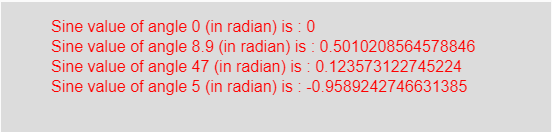# p5.js | sin() function

The sin() function in p5.js is used to calculate the sine value of an angle in radians taken as the input parameter of the function and gives the result in between -1 to 1.

Syntax:

`sin( angle )`

Parameters: This function accepts a single parameter angle which is an angle in radian whose sine value is calculated.

Return Value: It returns the sine value of an angle in radian taken as the input parameter.

Below program illustrates the sin() function in p5.js:

Example: This example uses sin() function to get sine value of an angle in radian.

 `function` `setup() {  ` `  `  `    ``// Create Canvas of size 270*80  ` `    ``createCanvas(550, 130);  ` `}  ` `  `  `function` `draw() {  ` `      `  `    ``// Set the background color  ` `    ``background(220);  ` `      `  `    ``// Initialize the parameter with ` `    ``// angles in radian only ` `    ``let a = 0;  ` `    ``let b = 8.9;  ` `    ``let c = 47; ` `    ``let d = 5; ` `      `  `    ``// Call to sin() function  ` `    ``let v = sin(a); ` `    ``let w = sin(b); ` `    ``let x = sin(c); ` `    ``let y = sin(d); ` `      `  `    ``// Set the size of text  ` `    ``textSize(16);  ` `      `  `    ``// Set the text color  ` `    ``fill(color(``'red'``));  ` `    `  `    ``// Getting sine value  ` `    ``text(``"Sine value of angle 0 (in radian) is : "` `+ v, 50, 30); ` `    ``text(``"Sine value of angle 8.9 (in radian) is : "` `+ w, 50, 50); ` `    ``text(``"Sine value of angle 47 (in radian) is : "` `+ x, 50, 70); ` `    ``text(``"Sine value of angle 5 (in radian) is : "` `+ y, 50, 90);      ` `}  `

Output:Note: In the above code, the input angle should be in radian. For converting the degree into radian use the following formula:

`Angles_in_radian = (π/180)*angles_in_degree`

Reference: https://p5js.org/reference/#/p5/sin

My Personal Notes arrow_drop_upCheck out this Author's contributed articles.

If you like GeeksforGeeks and would like to contribute, you can also write an article using contribute.geeksforgeeks.org or mail your article to contribute@geeksforgeeks.org. See your article appearing on the GeeksforGeeks main page and help other Geeks.

Please Improve this article if you find anything incorrect by clicking on the "Improve Article" button below.

Article Tags :

Be the First to upvote.

Please write to us at contribute@geeksforgeeks.org to report any issue with the above content.